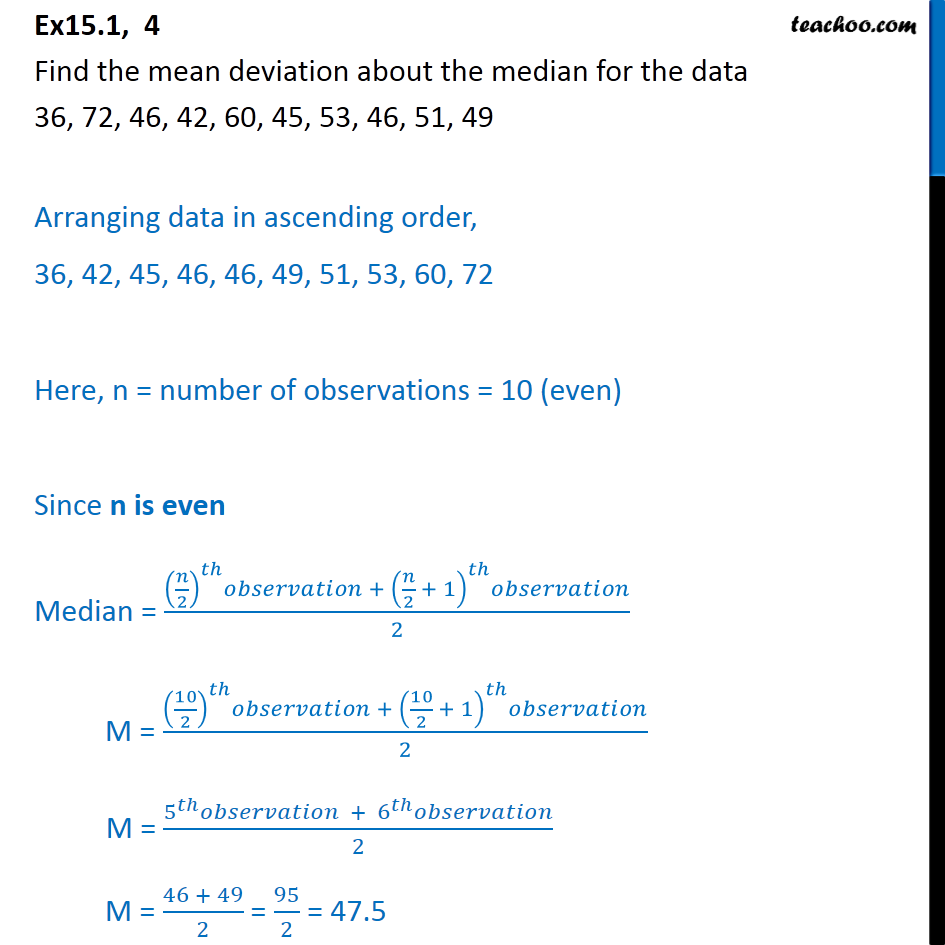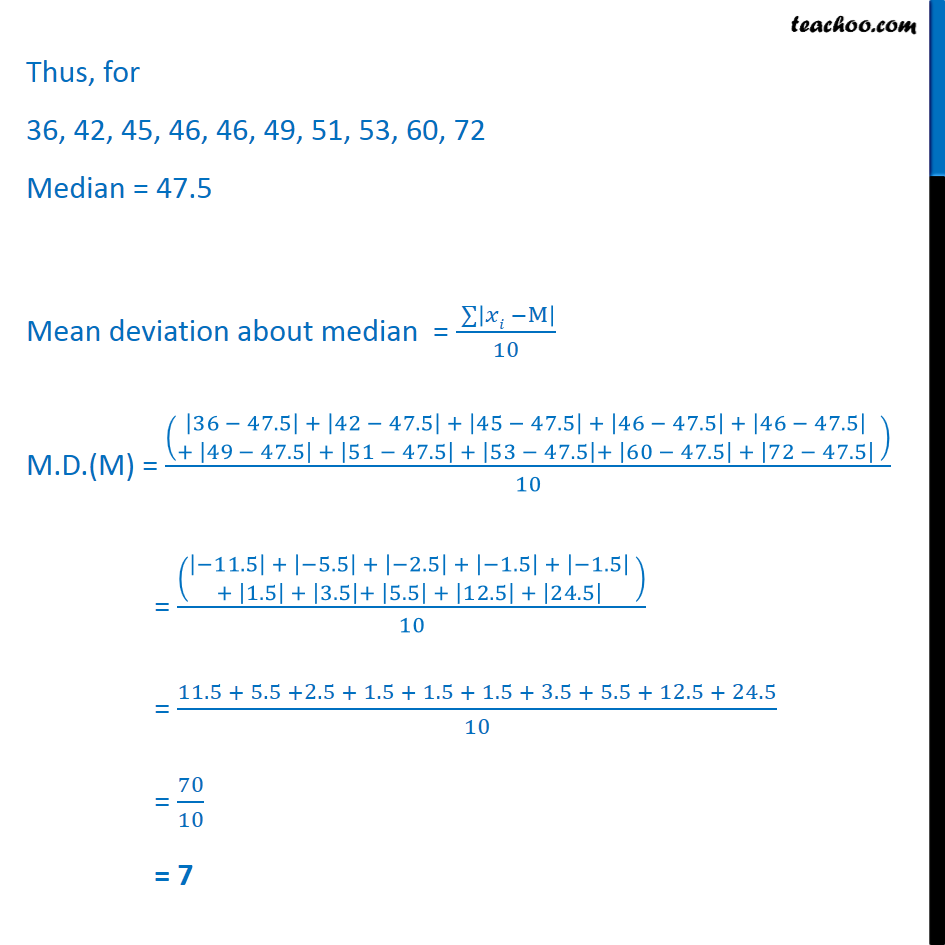Ex 13.1

Chapter 13 Class 11 Statistics
Serial order wiseLearn in your speed, with individual attention - Teachoo Maths 1-on-1 Class

### Transcript

Ex15.1, 4 Find the mean deviation about the median for the data 36, 72, 46, 42, 60, 45, 53, 46, 51, 49 Arranging data in ascending order, 36, 42, 45, 46, 46, 49, 51, 53, 60, 72 Here, n = number of observations = 10 (even) Since n is even Median = (( /2)^ + ( /2 + 1)^ )/2 M = ((10/2)^ + (10/2 + 1)^ )/2 M = (5^ + 6^ )/2 M = (46 + 49)/2 = 95/2 = 47.5 Thus, for 36, 42, 45, 46, 46, 49, 51, 53, 60, 72 Median = 47.5 Mean deviation about median = ( 128 | M| )/10 M.D.(M) = (( (|36 47.5| + |42 47.5| + |45 47.5| + |46 47.5| + |46 47.5| @+ |49 47.5| + |51 47.5| + |53 47.5|+ |60 47.5| + |72 47.5| )))/10 = (( (| 11.5| + | 5.5| + | 2.5| + | 1.5| + | 1.5| @+ |1.5| + |3.5|+ |5.5| + |12.5| + |24.5| )))/10 = (11.5 + 5.5 +2.5 + 1.5 + 1.5 + 1.5 + 3.5 + 5.5 + 12.5 + 24.5)/10 = 70/10 = 7